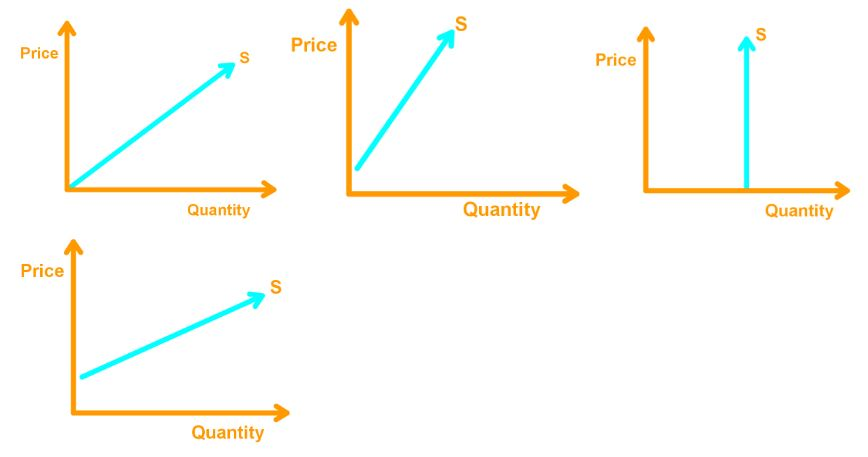# Elasticity of supply#### Everything You Need in One Place

Homework problems? Exam preparation? Trying to grasp a concept or just brushing up the basics? Our extensive help & practice library have got you covered.#### Learn and Practice With Ease

Our proven video lessons ease you through problems quickly, and you get tonnes of friendly practice on questions that trip students up on tests and finals.#### Instant and Unlimited Help

Our personalized learning platform enables you to instantly find the exact walkthrough to your specific type of question. Activate unlimited help now!##### Intros
###### Lessons
1. Elasticity of Supply Overview:
2. Elasticity of Supply
• Ratio of change in quantities supplied and change in price
• Formula for Elasticity of Supply
3. Types of Supply Curve
• Perfectly Inelastic
• Unit Elastic
• Perfectly Elastic
4. Factors which Influences Elasticity of Supply
• Resource Substitution
• Time Period for Supply
##### Examples
###### Lessons
1. Understanding Supply Elasticity of Supply
Suppose the percentage change in quantity supplied is 0% when the price increases. What is the elasticity of supply? Draw a supply curve that accurately represents this elasticity of supply.
1. Using the information in the table, find the elasticity of supply when the price rises from $50 to$60. Is it inelastic, or elastic? Does the supply curve intersect the origin?
 Price (dollars per bar chocolate bar) Quantity supplied (Chocolate Bars) 30 20 40 25 50 30 60 35 70 40
1. Which of the following supply curves accurately represents monetary supply for fruits and vegetables? Explain.1. Which of the following supply curves accurately represents long-run supply for fruits and vegetables? Explain.###### Topic Notes

Elasticity of Supply

To measure the responsiveness of quantities supplied to a change in the price of a good, we use the elasticity of supply.

In other words,

Elasticity of Supply = $\frac{\% \;change \;in \;quantity \;supplied}{\% \;change \;in \;price \;of \;quantity}$

To calculate the elasticity of supply, we use the following formula:

Elasticity of Supply = $\frac{(Q_{2}-Q_{1})/Q_{_{avg}}}{(P_{2}-P_{1})/P_{_{avg}}}$

Types of Supply Curves

There are three types of supply curves which have different elasticity of supply.

Case 1: Perfectly Inelastic

The supply curve is vertical and has an elasticity of supply of 0.In this case, a change in price leaves the quantity of supplied the same.

Case 2: Unit Elastic

The supply curve passes through the origin and is linear. The elasticity of supply is 1. Note that the slope of the curve does not matte. The elasticity of supply will always be 1.Case 3: Perfectly Elastic

The supply curve is horizontal, and the elasticity of supply is $\infty$Factors Which Influences Elasticity of Supply

Resource Substitution: If we can substitute resources to produce a good or service, then the elasticity of supply will be higher. With a small increase in price change, suppliers will produce more because they have more resource.

Time Period for Supply: The longer time passes after a price change, the greater the elasticity of supply is.

Momentary Supply: The longer time passes after a price change, the greater the elasticity of supply is.

Short-run Supply: When there is a price change, and we want to look at small adjustments that changes the quantity supplied, then we look at the short-run supply. Short-run supply is somewhat elastic.

Long-run Supply: When there is a price change, and we want to look at all possible adjustments that changes the quantity supplied, then we look at the long-run supply. Long-run supply is the most elastic.